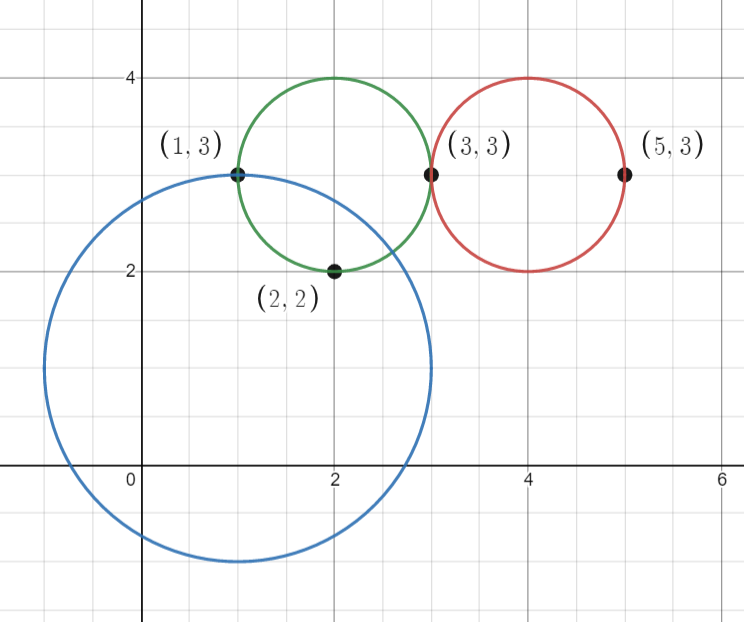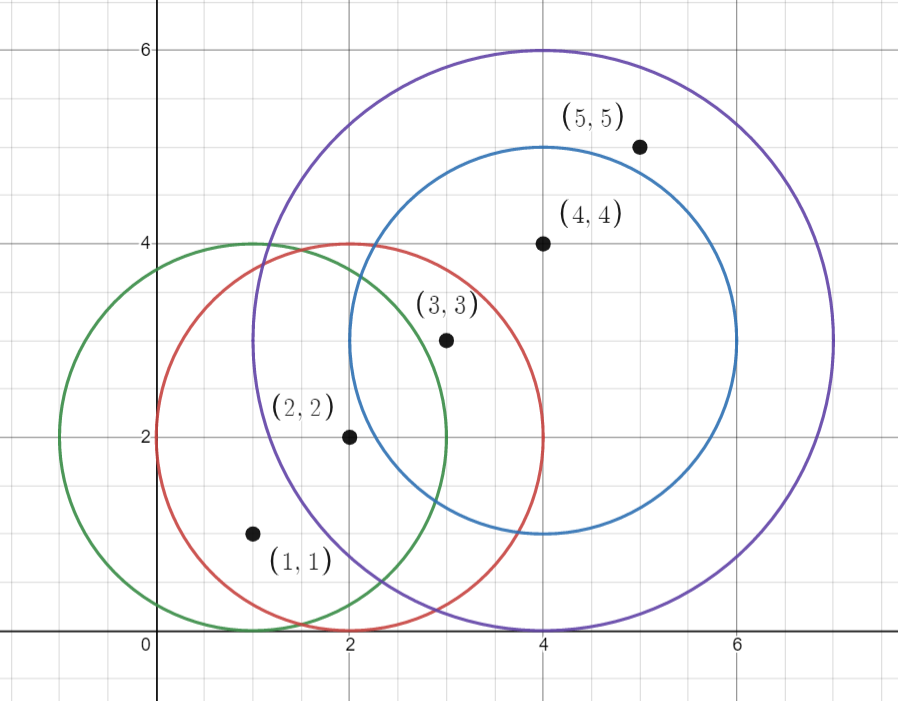1828. Queries on Number of Points Inside a Circle

You are given an array `points` where `points[i] = [xi, yi]` is the coordinates of the `ith` point on a 2D plane. Multiple points can have the same coordinates.

You are also given an array `queries` where `queries[j] = [xj, yj, rj]` describes a circle centered at `(xj, yj)` with a radius of `rj`.

For each query `queries[j]`, compute the number of points inside the `jth` circle. Points on the border of the circle are considered inside.

Return an array `answer`, where `answer[j]` is the answer to the `jth` query.

Example 1:```Input: points = [[1,3],[3,3],[5,3],[2,2]], queries = [[2,3,1],[4,3,1],[1,1,2]]
Output: [3,2,2]
Explanation: The points and circles are shown above.
queries is the green circle, queries is the red circle, and queries is the blue circle.
```

Example 2:```Input: points = [[1,1],[2,2],[3,3],[4,4],[5,5]], queries = [[1,2,2],[2,2,2],[4,3,2],[4,3,3]]
Output: [2,3,2,4]
Explanation: The points and circles are shown above.
queries is green, queries is red, queries is blue, and queries is purple.
```

Constraints:

• `1 <= points.length <= 500`
• `points[i].length == 2`
• `0 <= x​​​​​​i, y​​​​​​i <= 500`
• `1 <= queries.length <= 500`
• `queries[j].length == 3`
• `0 <= xj, yj <= 500`
• `1 <= rj <= 500`
• All coordinates are integers.

Follow up: Could you find the answer for each query in better complexity than `O(n)`?

1828. Queries on Number of Points Inside a Circle
``````struct Solution;

impl Solution {
fn count_points(points: Vec<Vec<i32>>, queries: Vec<Vec<i32>>) -> Vec<i32> {
queries.into_iter().map(|q| query(&points, q)).collect()
}
}

fn query(points: &[Vec<i32>], q: Vec<i32>) -> i32 {
let r2 = q * q;
let mut res = 0;
for p in points {
let dx = p - q;
let dy = p - q;
if dx * dx + dy * dy <= r2 {
res += 1;
}
}
res
}

#[test]
fn test() {
let points = vec_vec_i32![[1, 3], [3, 3], [5, 3], [2, 2]];
let queries = vec_vec_i32![[2, 3, 1], [4, 3, 1], [1, 1, 2]];
let res = vec![3, 2, 2];
assert_eq!(Solution::count_points(points, queries), res);

let points = vec_vec_i32![[1, 1], [2, 2], [3, 3], [4, 4], [5, 5]];
let queries = vec_vec_i32![[1, 2, 2], [2, 2, 2], [4, 3, 2], [4, 3, 3]];
let res = vec![2, 3, 2, 4];
assert_eq!(Solution::count_points(points, queries), res);
}
``````